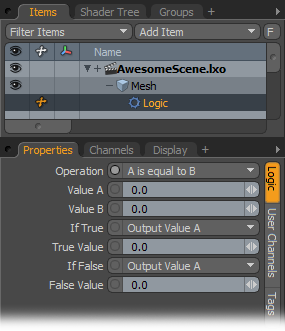# Conditional ModifiersWith the Logic modifier, you apply logic to channel operations.

Operation: You can select from the various logic operation options available in this list:

A is equal to B

A is greater then B

A is greater than or equal to B

A is less than B

A is less than or equal to B

A is not equal to B

And (The result is True if both A and B are not zero.)

Or (The result is True if A or B are not zero.)

Value A/Value B: These indicate the values of A or B for the operation. Typically you connect the output of another item's channel to these channels for the logic operation.

If True: Specify what Modo outputs when the logic modifier is True:

Output Value A

Output Value B

Output True Value as specified below

True Value: Specify the value to use if you select Output True Value.

If False: Specify what Modo outputs when the logic modifier is False.

Output Value A

Output Value B

Output False Value as specified below

False Value: Specify the value to use if you select Output False Value.